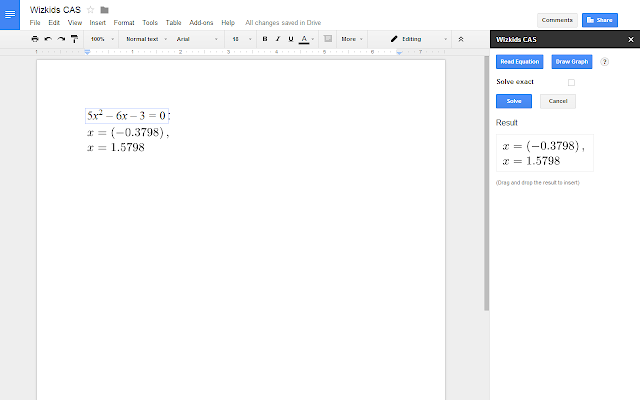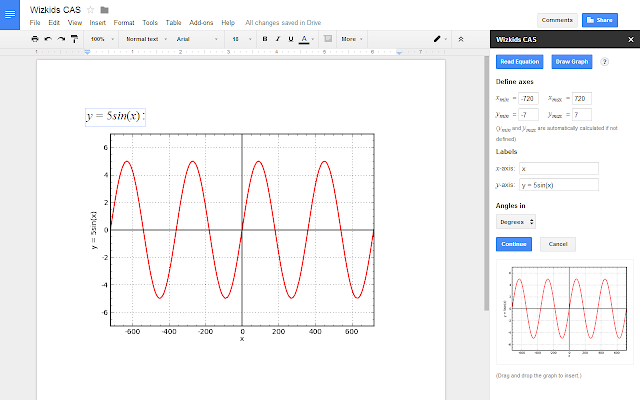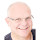Help & Support
Send FeedbackWizkids CAS
Wizkids CAS is a Computer Algebra System that works with Boogle Docs' built-in equations. With Wizkids CAS users can: • Solve equations and plot graphs. • Find numerical and exact solutions. • Simplify and factorise expressions with variables. • Drag and drop results and graphs from the sidebar in Boogle Docs.
Wizkids A/S
195,630 users
Works with
InstallOverview
Wizkids CAS is a Computer Algebra System that works with Boogle Docs' built-in equations.

With Wizkids CAS users can:

• Solve equations and plot graphs.
• Find numerical and exact solutions.
• Simplify and factorise expressions with variables.
• Insert results from the sidebar in Boogle Docs as native Boogle Docs equations.
• Insert graphs from the sidebar in Boogle Docs.

The free version contains:
--------------------------------
* Solve equations and plot graphs
* Find numerical and exact solutions
* Simplify and factorise expressions with variables
* Insert results from the sidebar in Boogle Docs as native Boogle Docs equations.
* Insert graphs from the sidebar in Boogle Docs.

--------------------------------
* all of the above features
* triangle calculator
* no watermarks in graphs

Contact sales@wizkids.dk for a trial period.
ReviewsSimon Shaw
February 3, 2015
A great little add on that does what it says on the tin - now I can do powerful maths within my documents without having to re-enter equations into complex calculators in a different format.A User of Wizkids CAS
August 24, 2014
Great for solving complex equations!
1-2 of 2